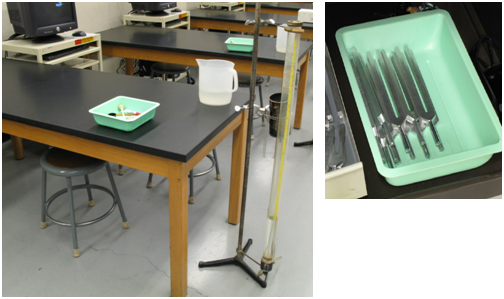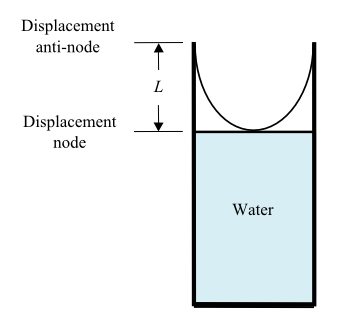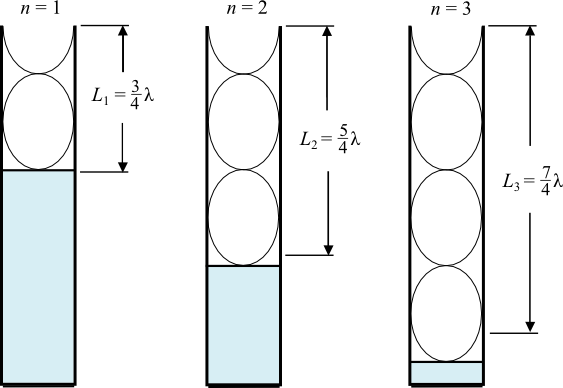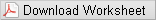# Speed of Sound - Resonance Tube

## Objectives

• to determine the speed of sound of waves in air

## Equipment

• resonance tube
• tuning forks
• rubber mallet
• measuring tape
• thermometerFigure 1

## Introduction and Theory

A sound wave is a longitudinal wave in which the wave oscillates along the direction of propagation. For a traveling wave of speed v, frequency f, and wavelength λ, the following relationship holds.
( 1 )
v = fλ
In this lab, we are going to use a simple characteristic of the traveling wave — the resonance — to determine the wavelength (and therefore the speed) of a sound wave. Consider a sound wave traveling through a resonance tube as illustrated in fig. 2.Figure 2: Resonance tube

A tuning fork is held by hand just above the open end of the tube. When the tuning fork is struck by a rubber hammer, it vibrates and sound waves are generated. These sound waves travel down the tube and are reflected upon reaching the surface of the water. The incoming and reflected waves interfere and form standing waves. The sound waves reflected from the water surface change their phase by 180° and therefore are completely out of phase with the incident sound waves. In other words, the amplitude of the standing waves must be zero at the water's surface. This point in space is usually referred to as a node. If a resonance condition is met, the open end of the tube has maximum amplitude of standing sound waves and is called an anti-node. At constant temperature the speed of sound is fixed; in addition, for a given tuning fork the frequency is also fixed, then according to eqn. 1, the wavelength of the sound wave should also be fixed. As a result the resonance conditions can only be satisfied when the length of the tube L is such that
( 2 )
Ln =
 1 4
(2n + 1)λ
where n = 0, 1, 2, 3, 4,..., and the length Ln is defined to be the distance measured from the open end of the tube to the water surface. For the specific example given in fig. 2 (n = 0), the length of the tube is
L =
 1 4
λ
which follows from eqn. 2. Fig. 3 shows resonance conditions in which n = 1, 2, and 3. It is easy to notice that
L2L1 =
 1 2
λ
L3L2 =
 1 2
λ
It can be predicted that the next resonance will occur at
L4 =
 7 4
λ +
 1 2
λ =
 9 4
λ
In other words:
( 3 )
Ln + 1Ln =
 1 2
λ
( 4 )
ΔL =
 1 2
λ
This relationship between the two consecutive resonances will be used to find the wavelength of the standing sound wave. The objective of this lab is to measure the speed of a sound wave in the air and compare it to its theoretical value.Figure 3: Examples of resonance for n = 1, 2, and 3.

According to the theory, the speed of sound in air depends upon the temperature of the air through the following relationship.
( 5 )
vT = (331.5 + 0.606T) m/s
Here, T is the temperature in centigrade (degrees Celsius). To achieve the objective TA will provide you with two tuning forks of known frequencies f. Remember to return both tuning forks to the TA by the end of the section. Be sure you clean your working space after you complete the experiment.

## Procedure

Please print the worksheet for this lab. You will need this sheet to record your data.Watch the level of water in the side container to avoid overflowing. Be sure to clean up any spills.
1
You will be provided with two tuning forks of known frequencies f. Note that the frequencies of tuning forks are marked on them. Write them down in the Worksheet Lab 10.
2
Fill the tube with water to about 10 cm to the open end of the tube. The level of the water in the tube (= the length of the tube L) can be adjusted by moving the side bucket up and down in the vertical direction.
3
Strike the tuning fork with the rubber head of the mallet for forks with frequencies below
1000 Hz
or with the wooden head for forks with frequencies above 1000 Hz, and place it just above the open end of the tube. Neither the hammer nor the vibrating fork should touch the tube.
4
Find as many resonances as you can for tuning fork one. Repeat your measurements by increasing the water level in the tube. Measure the length of the air column for each resonance from the top edge of the tube. Find the difference in length (ΔL) between the two consecutive resonances to calculate the wavelength of the sound wave. Once the wavelength is determined, the speed of sound follows from eqn. 1 (Note the error in the measurement of the length is 1 mm).
5
Repeat step 4 for the other tuning fork with a different frequency. In the Lab report you will need to calculate the average of the two measured speeds of sound and compare to the theoretical value.

## Discussion Questions

What was the purpose of the experiment? What is the theoretical concept that explains the expected experimental results? Where are a node and an antinode occuring in the sound standing wave in this lab experimental set up? Predict the length of the air column where the next resonance will occur. How will the experimental speed change if the temperature in the room is higher? Do the experimental results for the speed of sound in air agree with what is predicted from eqn. 3 within the error? Are the experimental results accurate? Are they precise? Discuss the most probable sources of errors in your measurements. Discuss the major reason for high discrepancy if that's the case.